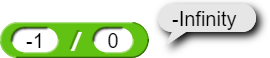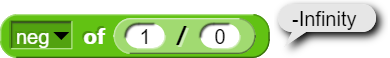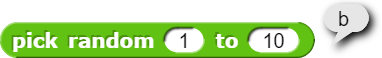# Are there any actual uses for this?

I have created a program that draws out a graph of numbers.
That's it in a nutshell.
Taking a random sample from this graph, you will find it is a number from 0-480, where the item's height is e^sqrt(x), where x is the number. I hope that's enough background?
(POSITIVE INFINITY is a reporter. Inside code is call(JS function(return Number.POSITIVE_INFINITY;)).)

I look at the data, and there is an eternal upwards trend (to be expected, numbers get bigger and bigger).
But now I am stuck with a question. What do I do with this data? What are use cases?? I created this program without knowing (well, I knew at one point and then forgot) what e even is, and now I have no idea what can be gleaned from the data.

Potentially necessary resources

EDIT: CODE ERROR! Example data is skewed and shouldn't be looked at! I forgot to change the / 5000 to / divisor. The project is fixed and normal, but the example data is old! Please run the project yourself to get new example data and see the project run. Apologies!

One neat fact about that is that the derivative of $$e^{x}$$ is $$e^{x}$$ itself, so the derivative of your graph is $$e^{\sqrt x}$$ is itself.

What is the derivative of something? I was quite literally just playing around with patterns when I discovered that e^x where x is anything creates some odd occurrences, so I decided to graph it.

The derivative of a function is the function that gives instantaneous rate of change of the original function at any particular point. (It's a calculus thing)

Correct me if I'm wrong: on a graph that represents a function that results in a sine, etc., the derivative is the slope at any given point?

Almost. The derivative is the function that gives what you said.

So, first of all, there's no need to use JS Function:To answer your question, I think your program confusingly does two things: graph a function and make a table of values of the function. Imho, graphing is super useful, for helping people understand the behavior of the function, but the table of values isn't so useful, unless the function takes a really long time to compute, because it's easier to compute values on the fly as needed than to maintain a table. Tables of values were essential before computers, and there were serious mathematicians who spent their whole life making such tables. But you don't know their names, because their work hasn't lived on. (And of course, being made by human beings, their tables were full of errors!)

Lists of values are important when the data are empirical (number of babies born in year $$x$$) rather than from computable functions.

This means you can make your graphing program much simpler, <10 blocks altogether. (And then you can complicate it again by auto-scaling the result, i.e., compute all the values and find their maximum, then make the plot dividing by that maximum. But you don't want to do that when the function's max value is infinite! :~) )

I don't really think that does answer my question. I do know that this project could easily graph with a much lower block count, but it is important to me to have it store data in a table, so I can do something else with the data. In the future (probably tomorrow) I will make a smaller version without the table-storing, but in the meantime, this is how I want my project to be.

That's interesting! I didn't know that. It's important to me that this infinity be positive infinity. Is 1/0 positive infinity???

Here, yes. If you want negative infinity, do (-1)/0:Or -(1/0):The first part of that is right, but not the second part. You've forgotten the chain rule:

$${{\rm d}f(g(x))\over{\rm d}x} = {{\rm d}f(g(x))\over{\rm d}g(x)}\cdot {{\rm d}g(x) \over {\rm d}x}$$

$${{\rm d}e^{\sqrt x}\over{\rm d}x} = {{\rm d}e^{\sqrt x}\over{\rm d}\sqrt x}\cdot {{\rm d}x^{1/2} \over {\rm d}x} = e^{\sqrt x} \cdot {{x^{-1/2}}\over{1/2}} = {2e^{\sqrt x} \over \sqrt x}$$

(Edit: From memory. If you want to be sure, ask Wolfram Alpha.)

I asked a college student and they said to remember chain rule???

EDIT: i am talking to said college student on discord and they literally sent this to me 5sec after bh posted-

Indeed I have.

Edit: I'm dumb. You already gave the formula.

What do you mean, "here"? 1/0 is infinite, anywhere.

Not in math! There, it isn't infinity, but an undefined value.

completely offtopic but I don't know what category to make a topic for this in

Can anyone explain why I saw a "b" from PICK RANDOM?:(that's a custom block I made, but I'm talking about the primitive PICK RANDOM.)

Umm. 0/0 is undefined, because it could be any value. $$\forall x, 0\cdot x=0$$, so divide both sides by zero to get $$\forall x, {0 \over 0} = x$$.

But in the case of 1/0, not any value will do. For any finite $$x$$, $$0\cdot x = 0 < 1$$. So 1/0 has to be larger than any finite x. Hence, ∞.

Your high school math teachers don't want you to think that you can embed 1/0 in a larger expression, e.g., $$\sqrt{1/0}$$, so they lump it in with undefined. But if you study numerical analysis, you'll learn that sometimes you can embed an infinite value in a larger expression and get a meaningful result. But you're allowed to do that only after you go to grad school.

Yeah: There's a bug in your custom block. :~P

But the limit from below and the limit from above are different.

No, I saw it clicking on the primitive. I think my eyes were playing tricks on me, though.

Yes, that's true. But just as by convention $$\sqrt x$$ means the positive square root, by convention 1/0 is +∞ and -1/0 is −∞.

Edit: No, I was right the first time:

I made a shorter, non-table version, as suggested by @bh.